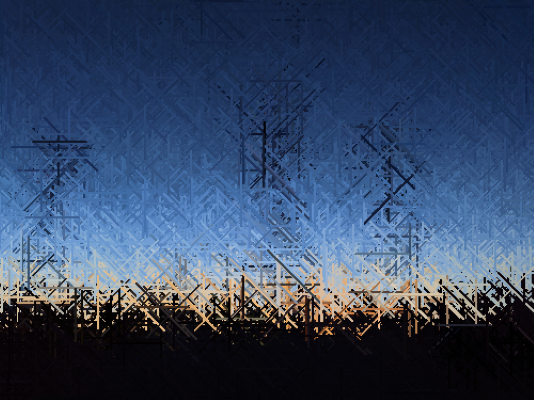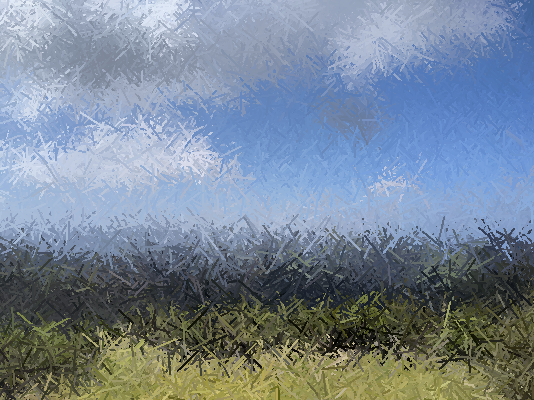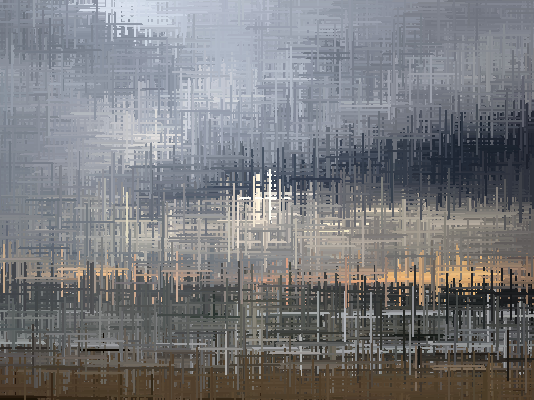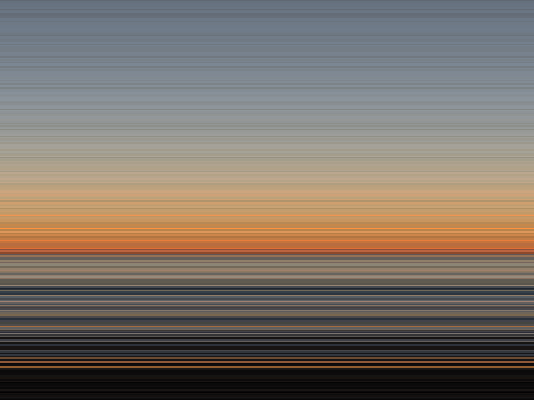# Glitch Lines

example processing images glitch-art genuary

Glitch art is art that incorporates bugs and imperfections to create interesting patterns. It was also the theme of Genuary 3, so I wrote this sketch that adds glitchy artifacts to an image.

``````int lineSize = 50;
PImage image;

void setup() {
size(500, 375);
image.resize(width, height);

image(image, 0, 0, width, height);

strokeWeight(2);
noSmooth();
}

void draw() {
for (int i = 0; i < 100; i++) {
drawOneLine();
}
}

void drawOneLine() {
int x = int(random(image.width));
int y = int(random(image.height));

color pixelColor = image.get(x, y);
stroke(pixelColor);

float r = random(1);

// Draw lines in 4 directions
if (r < .25) {
line(x - lineSize / 2, y, x + lineSize / 2, y);
} else if (r < .5) {
line(x, y - lineSize / 2, x, y + lineSize / 2);
} else if (r < .75) {
line(x - lineSize / 2, y - lineSize / 2,
x + lineSize / 2,
y + lineSize / 2);
} else {
line(x - lineSize / 2, y + lineSize / 2,
x + lineSize/2,
y - lineSize/2);
}

// Draw lines in random directions
// line(x, y,
//      x + random(-lineSize / 2, lineSize / 2),
//      y + random(-lineSize / 2, lineSize / 2));

// Draw lines in 2 directions
//if (r < .5){
//  line(0, y, width, y);
//} else {
//  line(x, 0, x, height);
//}
}
``````Here’s a coding timelapse of the above video:

# Remix Ideas

• Use this code on your own images.
• Change the logic for where the lines are drawn.
• Make parts of the image glitchier than others.